Basics of Geometry
edHelper subscribers - Create a new printable

Number of Keys
 Select the number of different printables: 1 key 2 keys 3 keys 4 keys 5 keys

Number of Pages (for each key)
 Select the number of pages: 1 page 2 pages 3 pages 4 pages

Include an answer key (answer keys will be at the end of the printable)
High School Geometry
High School Geometry
Math

 Name _____________________________Date ___________________ (Key 1 - Answer ID # 0229913)
1.

If you are sitting on a midpoint and the endpoints of the line are so far away you can't see them, what are some things you still know about the endpoints?

2.

Find a set of objects in the room such that each set contains something represented by one point, one line, and one plane. How many such sets are there?

3.

Which points are NOT coplanar with E?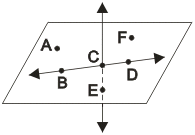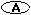A and F only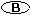B and D only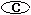A, B, D, and F only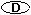A, B, C, D, and F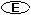All points are coplanar.

4.

Which points are collinear with I and Q?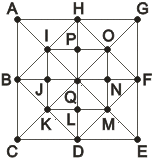C, G, K, and OB and HA, E, and MJ and PA, C, E, and G

5.

Is it possible for a bisector to divide a line into unequal parts? Explain.

6.

Do all books exhibit the same basic symmetry properties? Explain.

7.

Discuss the relationship between geometry and architecture.

8.

What is the difference between a point and an endpoint?

9.

Why are points, lines, and planes considered fundamental objects in geometry?

10.

Find a set of objects in the room such that each set contains one triangle, one circle, one square and one non-square rectangle.

Basics of Geometry
edHelper subscribers - Create a new printable

Number of Keys
 Select the number of different printables: 1 key 2 keys 3 keys 4 keys 5 keys

Number of Pages (for each key)
 Select the number of pages: 1 page 2 pages 3 pages 4 pages